Related Articles

# What are the Characteristics of Sound Waves?

• Last Updated : 20 May, 2021

Sound is nothing but the vibrations (a form of energy) that propagates in the form of waves through a certain medium. Different types of medium affect the properties of the wave differently. Does this mean that Sound will not travel if the medium does not exist? Correct. It will not, It is impossible for sound waves to travel in a vacuum. For different living beings, the audible frequency varies, for example, for humans, Sound waves that lie between the frequency of 20Hz to 20kHz are audible, All the frequencies lesser than this are infrasonic and all the frequencies above this frequency are ultrasonic for humans.

There are so many other characteristics of sound waves that affect the wave and its properties. Let’s take a look at them,

### Characteristics of Sound waves

Sound waves are those vibrations which when approaches our ears, are taken to the brain, and the brain then processes the information so that the sound is heard. The characteristics of sound waves affect the waves and the propagation in different ways, there are 5 basic characteristics of the sound wave. When the vibration is drawn in form of a wave, it becomes really easy to deduce the characteristics just by looking at the wave,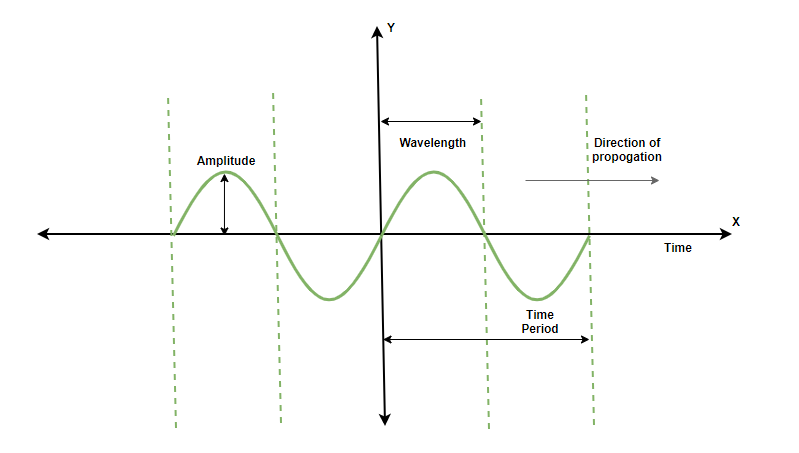Note: The wave shown above is a transverse wave, but sound waves are known to be longitudinal in nature, they represented in longitudinal form for better understanding of the concepts

Wavelength (λ)

A Wavelength is defined as the minimum distance covered by the Wave before it starts repeating itself. The wavelength is actually a length parameter, hence, the SI unit of wavelength is meter. The sound wave is longitude in nature, and the combination of the distance of one complete rarefaction and compression gives wavelength. The symbol for the wavelength used is λ.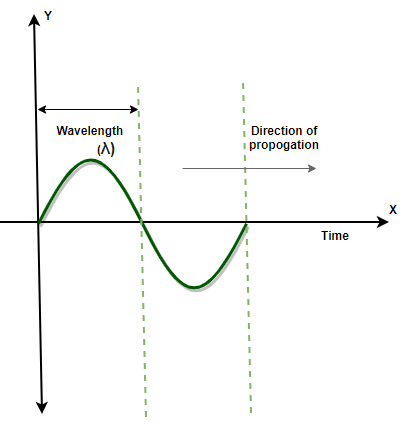Time Period (T)

The Time period of a sound wave is defined as the time required for one complete cycle. One cycle of the wave is defined by one complete rarefaction and compression in the vibration. The time period is measured in seconds. Time period is also used to find out the frequency of the wave, the reciprocal of the time period gives the frequency of the wave.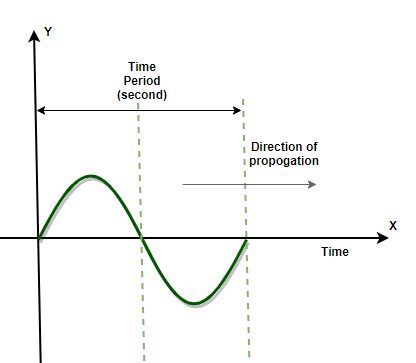Frequency/Pitch (ν)

The frequency of the sound wave is defined as the number of cycles completed by the wave in 1 second. The frequency of the wave can also be defined as the number of vibrations created by the wave in one second. The SI unit of frequency is Hertz (Hz) and it is represented as the symbol nu (ν). Frequency is that parameter of the wave which does not change by changing the medium of the propagation. Hence, the frequency of the wave always remains the same.

An example of the frequency of the wave is,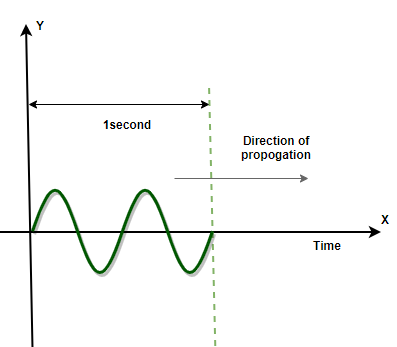In the above figure, the frequency of the wave is to be determined. As it is clearly visible that two cycles are completed in 1 second. Hence, it can be concluded that the frequency of the wave is 2 Hz.

Formula to find out the frequency of the wave,

Frequency (ν) = 1/T, Where T= Time Period

Note: The Time period and frequency of a wave are reciprocal to each other and therefore, if a wave has more Time period, it will have less frequency and vice versa.

Velocity of the wave

The velocity of the wave is defined as the distance traveled by the sound wave per unit of time. The distance traveled is nothing but the wavelength of the wave. The SI unit of the velocity of the sound wave is meter/second (m/sec).

Velocity of the sound wave = Wavelength/Time period

Velocity of the sound wave = Wavelength × Frequency

v = λ × ν (m/sec)

Amplitude/Loudness (A)

The Amplitude of the wave tells the amount of energy present in the wave. Amplitude is normally known as the loudness of the sound wave is defined by the maximum height (vertically) reached by the wave, the higher the magnitude, the higher will be the loudness. In a sound wave, the magnitude of one rarefaction or compression is defined as the amplitude of that wave.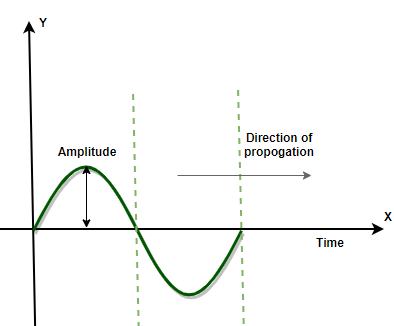### Sample Problems

Question 1: Explain with the help of diagrams how a certain sound is louder than the other?

The loudness of any sound is determined by its amplitude. Higher the amplitude of the wave, louder is the sound. Below given two different figures are showing the sound waves of two different sounds, where the first sound is louder and the second sound is less loud (quieter)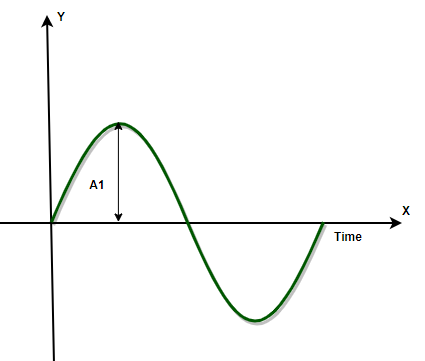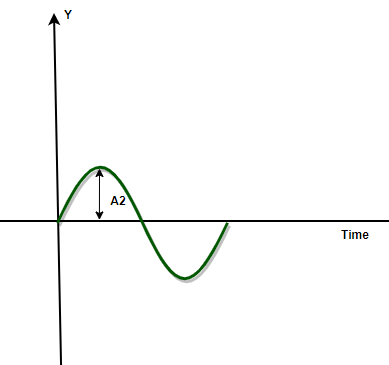Question 2: The Time period of a wave is given as 20 milliseconds. What will be the frequency of this wave?

As the formula known for the frequency of the wave is,

Frequency= 1/time period

Frequency= 1/(20 × 10-3)

Frequency= 1000/20

Frequency= 50Hz

Question 3: Two waves having Frequencies of 10Hz and 50Hz are given. Which wave has more Time period?

The Time period for the first wave= 1/frequency

= 1/10

= 0.1 second.

The Time period for the second wave= 1/frequency

= 1/50

=0.02 second.

Hence, The time period of the first wave more than the Time period of the second wave.

Question 4: Explain how the characteristics of a sound wave and a light wave differ from each other?

Difference between the characteristics of sound wave and light wave,

• Sound Waves are longitudinal waves while Light waves are transverse waves.
• Sound waves always require a medium to travel, that is, they cannot travel in vacuum, while light waves do not require any material medium since they are electromagnetic waves.
• The speed of light is approximately 330m/sec while the speed of light wave is 3× 108 m/sec.
• Sound waves are mechanical waves, but light waves are electromagnetic waves.

Question 5: The velocity of a wave is 300m/sec and the wavelength is given as 20Hz. What will be the wavelength of this wave?

The velocity of a wave is given as,

Velocity = frequency × wavelength

300= 20 × λ

λ =15meters

Hence, the wavelength of this wave is 15 meters.

Question 6: The Time period of a wave is 1 second and the wavelength is given as 10 meters. There is another wave with a time period of 3 seconds and the wavelength of 30 meters. Which of the two waves have more velocity.

Velocity of the first wave= 10/1

Velocity = 10m/sec

Velocity of the second wave= 30/3

Velocity = 10m/sec

Hence, the velocity of both the waves are equal.

Attention reader! Don’t stop learning now. Join the First-Step-to-DSA Course for Class 9 to 12 students , specifically designed to introduce data structures and algorithms to the class 9 to 12 students

My Personal Notes arrow_drop_up